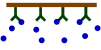### BIA-Pagesback
Affinity plot
back
back

# Affinity plot

The affinity plot shows the relation between the association (ka) and dissociation (kd) rate constants and the equilibrium dissociation constant (KD).

The association rate constants are on the y-axis in red. The dissociation rate constants are in blue on the x-axis. The intersections of the ka and kd are the equilibrium dissociation constant in green. The green lines connect the points with the same KD. The intermediate yellow box marks the range in which most of the association and dissociation rate constants can be expected. The four red dots show examples of the curves at the intersections (the analyte concentration is 1 nM = 1 x KD).

The affinity plot shows that the same equilibrium dissociation constant can have many different sensorgram shapes which depend on the rate constants. In addition the shape of the association curve is also dependent on the analyte concentration.

Compare the figure below with sensorgram B in the affinity plot. When the analyte concentration = 1x KD the curves look the same (scale is different). When the analyte concentration changes the association part changes but the dissociation part stays the same although due to the higher starting point the dissociation rate starts faster.

## Time to equilibrium

An important experimental consideration is the time taken to reach equilibrium. From the integrated rate equation for association, the time required (tΘ) to reach a fraction of equilibrium (Θ= R/Req) can be derived as:

To reach steady state faster it is possible to raise the analyte concentration, but this will not always work. Analyte concentrations above 100 times KD often lead to binding curves that are not an exponential and the bulk shift disturbs the curve significantly. It is better to increase the injection time. However, as shown in the table below, this can take a very long. The time to reach steady state mainly depends on the dissociation rate constant. Long injection times are not always feasible due to the limited amount of sample or the maximal injection volume.

Approximate calculated time required to reach 99.9% steady state at analyte concentrations ranging from 0.01 to 100 times KD.
concentration analytekd (s-1)
10-110-210-310-4
0.01 x KD68 s11.5 min115 min1140 min
0.1 x KD63 s10.5 min105 min1047 min
1 x KD34 s5.8 min57 min576 min
10 x KD6 s1 min10.5 min1105 min
100 x KD1 s0.1 min1 min11 min
ka = 1.105 M-1s-1